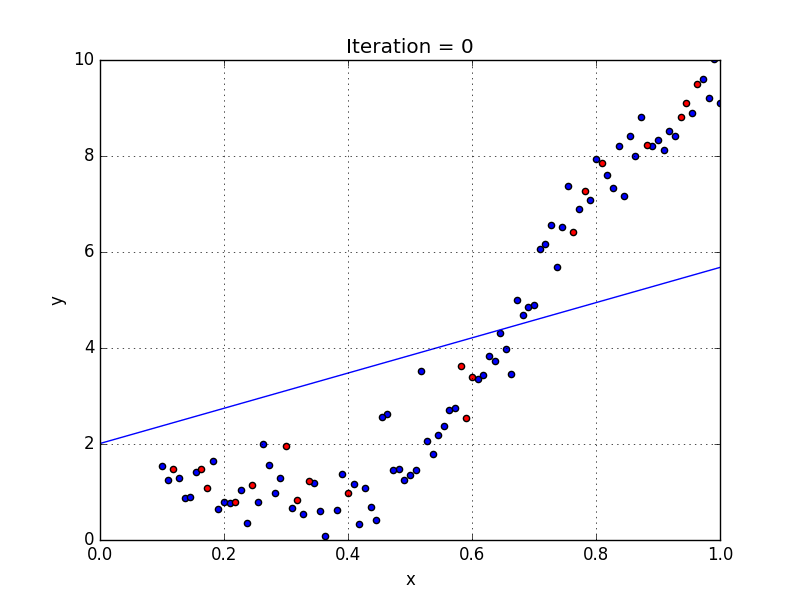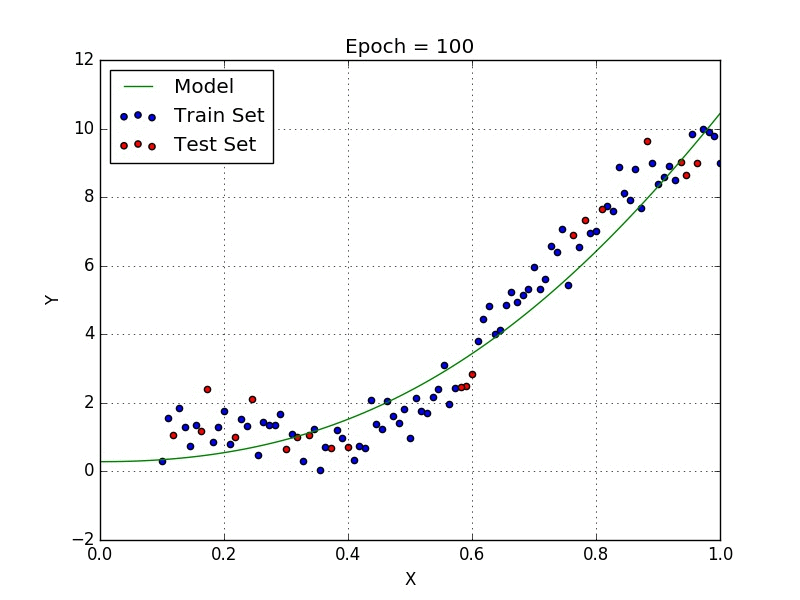top of page
Search

# Polynomial Regression along with code

Hello World, Today we are going to discuss Polynomial regression and I will make it a very easy topic for you. It is going to be an amazing tutorial. This article contains hands-on exercise of polynomial regression which will help us understand this topic more deeply.

Without any delay, Let's get started!

First thing first,

What is Regression?

Regression analysis is a form of predictive modeling technique that investigates the relationship between a dependent and independent variable.

The above-written definition is a bookish definition. If you understood the above line, it's well and good, If you didn't, it's OK.

Finding the best-fit regression equation using the relationship between dependent variable X and independent variable y, so that we can make predictions from it is known as regression.

Polynomial Regression

If you have seen my linear regression blog, it was having data that can fit into a straight line. But what if your data is actually more complex then a simple straight line? We can fit a polynomial regression model on that depending on how the data looks like.

What does polynomial regression do under the hood to fit the non-linear data?

Surprisingly, We can use a linear model to fit non-linear data. A simple method to do this is to add powers of each feature as new features then train a linear model on this extended set of features. This technique is known as polynomial regression.Figure 1: Linear Regression (source: Towards data science)Figure 2: Polynomial regression (source: Towards data science)

Therefore, if we have used a simple linear regression in the above graph as shown in figure 1 then as we can see the linear regression line would not fit the data very well. It is hard to fit a linear regression line on the graph of figure 1 and receiving a low error value. Hence, it is essential to fit a polynomial regression to achieve a minimum error.

General equation of Polynomial Function:-

Y=θo + θ₁X+ θ₂X² + … + θₘXᵐ +residual error

• The polynomial function gives the finest approximation of the relationship between its independent variable & dependent variable

• A wide range of curvature can be fit by the polynomial function

• It can fit a very broad range of functions

• The results of the non-linear analysis can be seriously affected by the presence of one or two outliers in the data

• There are only a few model validation tools for detecting the outliers in a Non-linear regression.

I conclude this article and take you to the coding section in Kaggle.